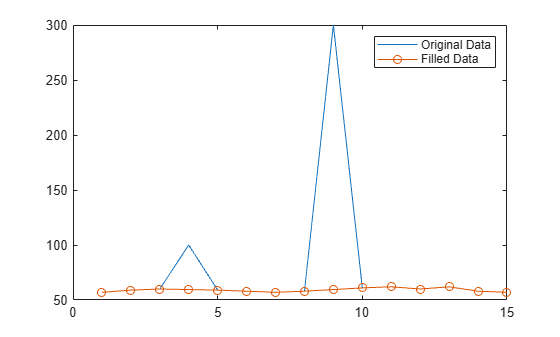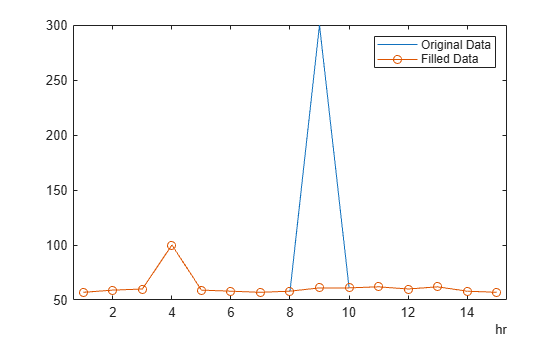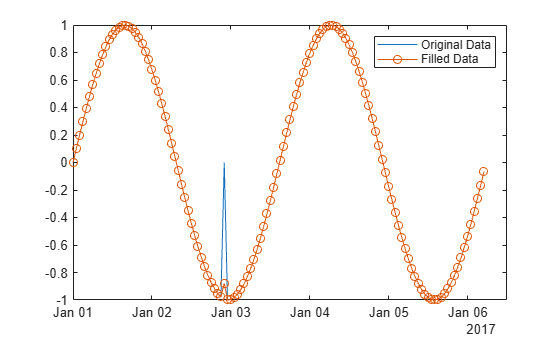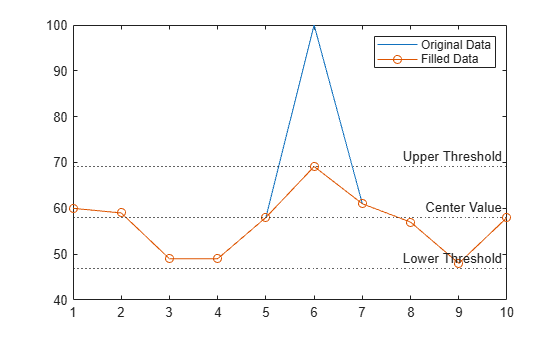Documentation

# filloutliers

Detect and replace outliers in data

## Syntax

``B = filloutliers(A,fillmethod)``
``B = filloutliers(A,fillmethod,findmethod)``
``B = filloutliers(A,fillmethod,'percentiles',threshold)``
``B = filloutliers(A,fillmethod,movmethod,window)``
``B = filloutliers(___,dim)``
``B = filloutliers(___,Name,Value)``
``````[B,TF,L,U,C] = filloutliers(___)``````

## Description

example

````B = filloutliers(A,fillmethod)` finds outliers in `A` and replaces them according to `fillmethod`. For example, `filloutliers(A,'previous')` replaces outliers with the previous non-outlier element. By default, an outlier is a value that is more than three scaled median absolute deviations (MAD) away from the median. If `A` is a matrix or table, then `filloutliers` operates on each column separately. If `A` is a multidimensional array, then `filloutliers` operates along the first dimension whose size does not equal 1.```

example

````B = filloutliers(A,fillmethod,findmethod)` specifies a method for detecting outliers. For example, `filloutliers(A,'previous','mean')` defines an outlier as an element of `A` more than three standard deviations from the mean.```
````B = filloutliers(A,fillmethod,'percentiles',threshold)` defines outliers as points outside of the percentiles specified in `threshold`. The `threshold` argument is a two-element row vector containing the lower and upper percentile thresholds, such as `[10 90]`.```

example

````B = filloutliers(A,fillmethod,movmethod,window)` specifies a moving method for detecting local outliers according to a window length defined by `window`. For example, `filloutliers(A,'previous','movmean',5)` identifies outliers as elements more than three local standard deviations away from the local mean within a five-element window.```

example

````B = filloutliers(___,dim)` operates along dimension `dim` of `A` for any of the previous syntaxes. For example, `filloutliers(A,'linear',2)` operates on each row of a matrix `A`.```

example

````B = filloutliers(___,Name,Value)` specifies additional parameters for detecting and replacing outliers using one or more name-value pair arguments. For example, `filloutliers(A,'previous','SamplePoints',t)` detects outliers in `A` relative to the corresponding elements of a time vector `t`.```

example

``````[B,TF,L,U,C] = filloutliers(___)``` also returns information about the position of the outliers and thresholds computed by the detection method. `TF` is a logical array indicating the location of the outliers in `A`. The `L`, `U`, and `C` arguments represent the lower and upper thresholds and the center value used by the outlier detection method.```

## Examples

collapse all

Create a vector of data containing an outlier, and use linear interpolation to replace the outlier. Plot the original and filled data.

```A = [57 59 60 100 59 58 57 58 300 61 62 60 62 58 57]; B = filloutliers(A,'linear'); plot(1:15,A,1:15,B,'o') legend('Original Data','Interpolated Data')```Create a vector containing an outlier, and define outliers as points outside three standard deviations from the mean. Replace the outlier with the nearest element that is not an outlier, and plot the original data and the interpolated data.

```A = [57 59 60 100 59 58 57 58 300 61 62 60 62 58 57]; B = filloutliers(A,'nearest','mean'); plot(1:15,A,1:15,B,'o') legend('Original Data','Interpolated Data')```Use a moving median to find local outliers within a sine wave that corresponds to a time vector.

Create a vector of data containing a local outlier.

```x = -2*pi:0.1:2*pi; A = sin(x); A(47) = 0;```

Create a time vector that corresponds to the data in `A`.

`t = datetime(2017,1,1,0,0,0) + hours(0:length(x)-1);`

Define outliers as points more than three local scaled MAD away from the local median within a sliding window. Find the location of the outlier in `A` relative to the points in `t` with a window size of 5 hours. Fill the outlier with the computed threshold value using the method `'clip'`, and plot the original and filled data.

```[B,TF,U,L,C] = filloutliers(A,'clip','movmedian',hours(5),'SamplePoints',t); plot(t,A,t,B,'o') legend('Original Data','Filled Data')```Display the threshold value that replaced the outlier.

`L(TF)`
```ans = -0.8779 ```

Fill outliers for each row of a matrix.

Create a matrix of data containing outliers along the diagonal.

`A = randn(5,5) + diag(1000*ones(1,5))`
```A = 5×5 103 × 1.0005 -0.0013 -0.0013 -0.0002 0.0007 0.0018 0.9996 0.0030 -0.0001 -0.0012 -0.0023 0.0003 1.0007 0.0015 0.0007 0.0009 0.0036 -0.0001 1.0014 0.0016 0.0003 0.0028 0.0007 0.0014 1.0005 ```

Fill outliers with zeros based on the data in each row, and display the new values.

```[B,TF,lower,upper,center] = filloutliers(A,0,2); B```
```B = 5×5 0 -1.3077 -1.3499 -0.2050 0.6715 1.8339 0 3.0349 -0.1241 -1.2075 -2.2588 0.3426 0 1.4897 0.7172 0.8622 3.5784 -0.0631 0 1.6302 0.3188 2.7694 0.7147 1.4172 0 ```

You can directly access the detected outlier values and their filled values using `TF` as an index vector.

`[A(TF) B(TF)]`
```ans = 5×2 103 × 1.0005 0 0.9996 0 1.0007 0 1.0014 0 1.0005 0 ```

Find the outlier in a vector of data, and replace it using the `'clip'` method. Plot the original data, the filled data, and the thresholds and center value determined by the detection method. `'clip'` replaces the outlier with the upper threshold value.

```x = 1:10; A = [60 59 49 49 58 100 61 57 48 58]; [B,TF,lower,upper,center] = filloutliers(A,'clip'); plot(x,A,x,B,'o',x,lower*ones(1,10),x,upper*ones(1,10),x,center*ones(1,10)) legend('Original Data','Filled Data','Lower Threshold','Upper Threshold','Center Value')```## Input Arguments

collapse all

Input data, specified as a vector, matrix, multidimensional array, table, or timetable.

If `A` is a table, then its variables must be of type `double` or `single`, or you can use the `'DataVariables'` name-value pair to list `double` or `single` variables explicitly. Specifying variables is useful when you are working with a table that contains variables with data types other than `double` or `single`.

If `A` is a timetable, then `filloutliers` operates only on the table elements. Row times must be unique and listed in ascending order.

Data Types: `double` | `single` | `table` | `timetable`

Fill method for replacing outliers, specified as a numeric scalar or one of the following:

Fill MethodDescription
Numeric scalarFills with specified scalar value
`'center'`Fills with the center value determined by `findmethod`
`'clip'`Fills with the lower threshold value for elements smaller than the lower threshold determined by `findmethod`. Fills with the upper threshold value for elements larger than the upper threshold determined by `findmethod`
`'previous'`Fills with the previous non-outlier value
`'next'`Fills with the next non-outlier value
`'nearest'`Fills with the nearest non-outlier value
`'linear'`Fills using linear interpolation of neighboring, non-outlier values
`'spline'`Fills using piecewise cubic spline interpolation
`'pchip'`Fills using shape-preserving piecewise cubic spline interpolation
`'makima'`modified Akima cubic Hermite interpolation (numeric, `duration`, and `datetime` data types only)

Data Types: `double` | `single` | `char`

Method for detecting outliers, specified as one of the following:

MethodDescription
`'median'`Outliers are defined as elements more than three scaled MAD from the median. The scaled MAD is defined as `c*median(abs(A-median(A)))`, where `c=-1/(sqrt(2)*erfcinv(3/2))`.
`'mean'`Outliers are defined as elements more than three standard deviations from the mean. This method is faster but less robust than `'median'`.
`'quartiles'`Outliers are defined as elements more than 1.5 interquartile ranges above the upper quartile (75 percent) or below the lower quartile (25 percent). This method is useful when the data in `A` is not normally distributed.
`'grubbs'`Outliers are detected using Grubbs’s test, which removes one outlier per iteration based on hypothesis testing. This method assumes that the data in `A` is normally distributed.
`'gesd'`Outliers are detected using the generalized extreme Studentized deviate test for outliers. This iterative method is similar to `'grubbs'`, but can perform better when there are multiple outliers masking each other.

Percentile thresholds, specified as a two-element row vector whose elements are in the interval [0,100]. The first element indicates the lower percentile threshold and the second element indicates the upper percentile threshold. For example, a threshold of `[10 90]` defines outliers as points below the 10th percentile and above the 90th percentile. The first element of `threshold` must be less than the second element.

Moving method for detecting outliers, specified as one of the following:

MethodDescription
`'movmedian'`Outliers are defined as elements more than three local scaled MAD from the local median over a window length specified by `window`.
`'movmean'`Outliers are defined as elements more than three local standard deviations from the local mean over a window length specified by `window`.

Window length, specified as a positive integer scalar, a two-element vector of positive integers, a positive duration scalar, or a two-element vector of positive durations.

When `window` is a positive integer scalar, the window is centered about the current element and contains `window-1` neighboring elements. If `window` is even, then the window is centered about the current and previous elements.

When `window` is a two-element vector of positive integers `[b f]`, the window contains the current element, `b` elements backward, and `f` elements forward.

When `A` is a timetable or `'SamplePoints'` is specified as a `datetime` or `duration` vector, `window` must be of type `duration`, and the windows are computed relative to the sample points.

Data Types: `double` | `single` | `int8` | `int16` | `int32` | `int64` | `uint8` | `uint16` | `uint32` | `uint64` | `duration`

Dimension to operate along, specified as a positive integer scalar. If no value is specified, then the default is the first array dimension whose size does not equal 1.

Consider a matrix `A`.

`filloutliers(A,fillmethod,1)` fills outliers according to the data in each column.`filloutliers(A,fillmethod,2)` fills outliers according to the data in each row.When `A` is a table or timetable, `dim` is not supported. `filloutliers` operates along each table or timetable variable separately.

Data Types: `double` | `single` | `int8` | `int16` | `int32` | `int64` | `uint8` | `uint16` | `uint32` | `uint64`

### Name-Value Pair Arguments

Specify optional comma-separated pairs of `Name,Value` arguments. `Name` is the argument name and `Value` is the corresponding value. `Name` must appear inside quotes. You can specify several name and value pair arguments in any order as `Name1,Value1,...,NameN,ValueN`.

Example: `filloutliers(A,'center','mean','ThresholdFactor',4)`

Detection threshold factor, specified as the comma-separated pair consisting of `'ThresholdFactor'` and a nonnegative scalar.

For methods `'median'` and `'movmedian'`, the detection threshold factor replaces the number of scaled MAD, which is 3 by default.

For methods `'mean'` and `'movmean'`, the detection threshold factor replaces the number of standard deviations from the mean, which is 3 by default.

For methods `'grubbs'` and `'gesd'`, the detection threshold factor is a scalar ranging from 0 to 1. Values close to 0 result in a smaller number of outliers and values close to 1 result in a larger number of outliers. The default detection threshold factor is 0.5.

For the `'quartiles'` method, the detection threshold factor replaces the number of interquartile ranges, which is 1.5 by default.

This name-value pair is not supported when the specified method is `'percentiles'`.

Data Types: `double` | `single` | `int8` | `int16` | `int32` | `int64` | `uint8` | `uint16` | `uint32` | `uint64`

Sample points, specified as the comma-separated pair consisting of `'SamplePoints'` and a vector. The sample points represent the location of the data in `A`, and must be sorted and contain unique elements. Sample points do not need to be uniformly sampled. If `A` is a timetable, then the default sample points vector is the vector of row times. Otherwise, the default vector is `[1 2 3 ...]`.

Moving windows are defined relative to the sample points. For example, if `t` is a vector of times corresponding to the input data, then `filloutliers(rand(1,10),'previous','movmean',3,'SamplePoints',t)` has a window that represents the time interval between `t(i)-1.5` and `t(i)+1.5`.

When the sample points vector has data type `datetime` or `duration`, then the moving window length must have type `duration`.

Data Types: `single` | `double` | `datetime` | `duration`

Table variables, specified as the comma-separated pair consisting of `'DataVariables'` and a variable name, a cell array of variable names, a numeric vector, a logical vector, or a function handle. The `'DataVariables'` value indicates which columns of the input table to detect outliers in, and can be one of the following:

• A character vector specifying a single table variable name

• A cell array of character vectors where each element is a table variable name

• A vector of table variable indices

• A logical vector whose elements each correspond to a table variable, where `true` includes the corresponding variable and `false` excludes it

• A function handle that takes the table as input and returns a logical scalar

Example: `'Age'`

Example: `{'Height','Weight'}`

Example: `@isnumeric`

Data Types: `char` | `cell` | `double` | `single` | `logical` | `function_handle`

Maximum outlier count, for the `'gesd'` method only, specified as the comma-separated pair consisting of `'MaxNumOutliers'` and a positive scalar. The `'MaxNumOutliers'` value specifies the maximum number of outliers returned by the `'gesd'` method. For example, `filloutliers(A,'linear','gesd','MaxNumOutliers',5)` returns no more than five outliers.

The default value for `'MaxNumOutliers'` is the integer nearest to 10 percent of the number of elements in `A`. Setting a larger value for the maximum number of outliers can ensure that all outliers are detected, but at the cost of reduced computational efficiency.

Data Types: `double` | `single` | `int8` | `int16` | `int32` | `int64` | `uint8` | `uint16` | `uint32` | `uint64`

Known outlier indicator, specified as the comma-separated pair consisting of `'OutlierLocations'` and a logical vector, matrix, or multidimensional array of the same size as `A`. The known outlier indicator elements can be `true` to indicate an outlier in the corresponding location of `A` or `false` otherwise. Specifying `'OutlierLocations'` turns off the default outlier detection method, and uses only the elements of the known outlier indicator to define outliers.

The `'OutlierLocations'` name-value pair cannot be specified when `findmethod` is specified.

The output `TF` is the same as the `'OutlierLocations'` value.

Data Types: `logical`

## Output Arguments

collapse all

Filled outlier array, returned as a vector, matrix, multidimensional array, table, or timetable. The elements of `B` are the same as those of `A`, but with all outliers replaced according to `fillmethod`.

Data Types: `double` | `single` | `table` | `timetable`

Outlier indicator, returned as a vector, matrix, or multidimensional array. An element of `TF` is `true` when the corresponding element of `A` is an outlier and `false` otherwise. `TF` is the same size as `A`.

Data Types: `logical`

Lower threshold used by the outlier detection method, returned as a scalar, vector, matrix, multidimensional array, table, or timetable. For example, the lower value of the default outlier detection method is three scaled MAD below the median of the input data. `L` has the same size as `A` in all dimensions except for the operating dimension where the length is 1.

Data Types: `double` | `single` | `table` | `timetable`

Upper threshold used by the outlier detection method, returned as a scalar, vector, matrix, multidimensional array, table, or timetable. For example, the upper value of the default outlier detection method is three scaled MAD above the median of the input data. `U` has the same size as `A` in all dimensions except for the operating dimension where the length is 1.

Data Types: `double` | `single` | `table` | `timetable`

Center value used by the outlier detection method, returned as a scalar, vector, matrix, multidimensional array, table, or timetable. For example, the center value of the default outlier detection method is the median of the input data. `C` has the same size as `A` in all dimensions except for the operating dimension where the length is 1.

Data Types: `double` | `single` | `table` | `timetable`

## More About

collapse all

### Median Absolute Deviation

For a random variable vector A made up of N scalar observations, the median absolute deviation (MAD) is defined as

for i = 1,2,...,N.

The scaled MAD is defined as `c*median(abs(A-median(A)))` where `c=-1/(sqrt(2)*erfcinv(3/2))`.

Download ebook